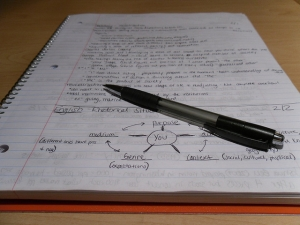FSc Part 2 Physics Numerical Chapter 12 Electrostatics

0
109

FSc Part 2 Physics Numerical Chapter 12 Electrostatics Pakistan BoardThe Solutions of these problems are given at the end in blue link.

Here is the Problems of Physics Part two Chapter 12.

Problem No 1:

Compare Magnitude of electrical and gravitational forces Exerted on an object (Mass = 10.0 g, Charge = 20.0 µC) by an Identical Object that is Placed 10.0 cm from the first. (G = 6.67 × 10-11 Nm2 kg-2)

Problem: 2

A point charge q = – 8.0 × 10-9 C is placed at the origin. Calculate electric field at a point 2.0 m from the origin on the z-axis.

Problem 3

Dtermine the electric field at position r = (4i + 3j)m caused by a point charge q = 5.0 × 10-6 C Placed at origin.

Problem 4

Two point charges, q1 = -1.0 × 10-6C and q1 = -1.0 × 10-6C, are separated by a distance of 3.0m. Find and justify the zero field location.

Problem 5

Find the electric field strength required to hold suspended a particle of mass 1.0 × 10-6 Kg and charge 1.0µC between two plates 10.0 cm apart.

Problem 6

A particle having a charge of 20 electrons on it falls through a potential difference of 100 volts. Calculate the energy acquired by it in electron volts (eV).

Problem 7

In the Millikan’s experiment, oil droplets are introduced into the space between two flat horizontal plates, 500 mm apart. The plate voltage is adjusted to exactly 780V so that the droplet is held stationary. The plate voltage is switched off t and he selected droplets is observed to fall a measured distance of 1.50 mm in 11.2 s. Given that the density of the oil used is 900kgm-3, and the viscosity of air at laboratory temperature is 1.80 × 10-5Nm-5s, calculate:

a)   the mass, and

b)   the charge on the droplet (assume g = 9.8 ms-2)

Problem: 8

A proton place in uniform electric field of 5000 NC-1 directed to right is allowed to go a distance of 10.0 cm from A to B. Calculate:

a)   potential difference between two points

b)   work done

c)    the change in P.E of proton

d)   the change in K.E of the proton

e)    Its velocity (mass of proton is 0.67 × 10-27)

Problem: 9

Using zero reference point at infinity, determine the amount by which a point charge of 4.0 × 10-8C alters the electric potential at a point 1.2 m away, when a) charge is positive    b) Charge is negative.

Problem: 10

In Bohr’s atomic model of hydrogen atom, the electron is in an orbit around the nuclear proton at a distance of 5.29  10-11 m with a speed of 2.18   106 ms-1.

a)   The electric potential that a proton exerts at this distance

b)   Total energy of the atom in eV

c)    The ionization energy for the atom in eV

Problem no: 11

The electronic flash attachment for a camera contains a capacitor for storing the energy used to produce the flash. In one such unit, the potential difference between the plates of a 750µF capacitor is 300 V. Determine the energy that is used to produce the flash.

Problem no: 12

A capacitor has a capacitance of 2.5 10-8 F. In the charging process, electrons are removed from one plate and placed on the other one. When the potential difference between the plates is 450 V, how many electrons have been transferred?

Only you have to download this link to Find the solutions of All numerical of Fsc Physics Part two Chapter 12.

The Solution and Answers of these Problems are given in the blue Link below:

FSC Part 2 Physics Numerical Chapter 12